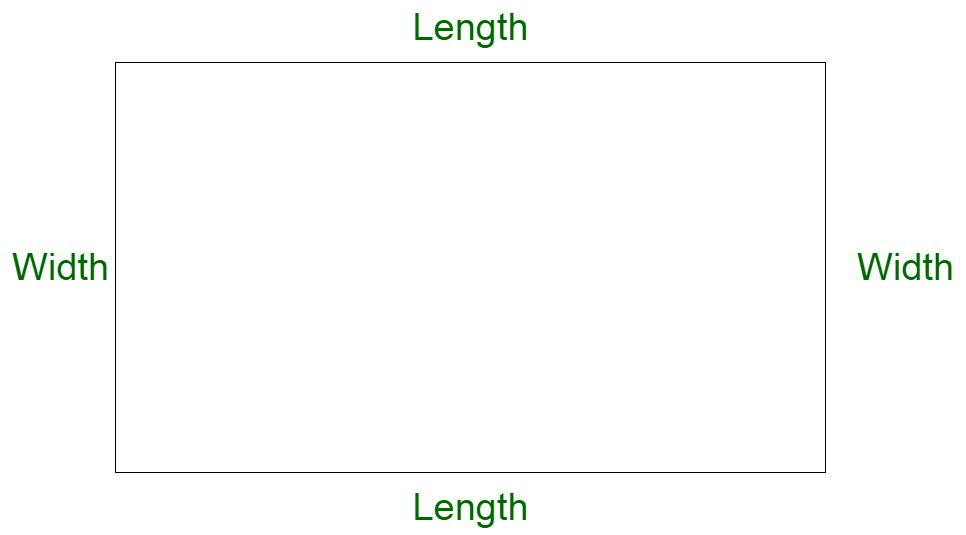GeeksforGeeks App
Open AppBrowser
Continue

# What happens to the area of a rectangle if its length is doubled and the breadth remaining the same?

In Mathematics, mensuration deals with geometric figures and parameters like volume, area, shape, surface area, etc. Or in other words, when we work with the area, the volume of specific shapes, or different parameters of geometric figures then it is called Mensuration in Mathematics.

Rectangle

A rectangle is a closed two-dimensional figure composed of four sides and four vertices. All angles of the rectangle are 90°. A rectangle with all sides equal is equivalent to a square. A rectangle is composed of two pairs of parallel sides, length, and width respectively.Properties of rectangle

• A rectangle has four sides with four angles.
• The angles of a rectangle are the right angles that are 90° each.
• The opposite sides of a rectangle are parallel and equal in length.
• The diagonals of the rectangle bisect each other and both the diagonals have the same length.
• The sum of all the interior angles of a rectangle is equal to 360°.

Area of Rectangle

A rectangle is composed of equal pairs which are parallel in nature and equal in length. The area of a rectangle is the space enclosed within its boundaries. Or in other words, the product of the length and width of the rectangle is known as the area of a rectangle.

Area of rectangle = Length x Width

Let us assume A to be the area of the rectangle and l and b to be the length and breadth of the rectangle respectively.

A = l x b

### What happens to the area of a rectangle if its length is doubled and the breadth remaining the same?

Solution:

Let us assume A to be the original area of the rectangle.

Let us assume l and b to be the length and breadth of the original rectangle respectively.

Now,

A = l x b

Now,

Let us assume l’ and b’ to be the length and breadth of the new rectangle respectively.

A’ = l’ x b’

Now, the length is doubled and breadth remains the same, therefore,

l’ = 2l

b’ = b

We get,

A’ = 2l x b

A’ = 2 (l x b)

A’ = 2A

Hence, when the length is doubled and the breadth remaining the same then the area of the rectangle becomes twice

### Sample Questions

Question 1: How does the area of the rectangle change when the length is doubled and breadth halved?

Solution:

We know,

Area of rectangle = length x breadth

Therefore,

A = l x b

Now,

l’ = 2l

b’ = b/2

Now, computing the area,

A’ = l’ x b’

A’ = 2l x b/2

A’ = l x b

Therefore, area remains same.

Question 2: How does the area change if length and breadth become equal?

Solution:

We know,

Area of rectangle = length x breadth

Therefore,

Area of rectangle = length x length

= (length)2

In this situation, the rectangle becomes a square.

Question 3: Derive the general formula of the change in the area of a rectangle if length becomes m times and breadth becomes n times.

Solution:

We know,

Area of rectangle, A = length x breadth

Therefore, in the modified case,

l ‘ = m x l

b’ = n x b

Area of rectangle, A’ =  l’ x b’

Area of rectangle, A’ =  m x l x n x b

Area of rectangle, A’ = m x n x (l x b)

A’ = m x n x A

Therefore, area becomes (m xn) times.

Question 4: Using the above formula, define how the area will change if length becomes 1/8 times and breadth 2 times.

Solution:

Area of rectangle = length x breadth

A’ = 1/8 x 2 A

= 1/4 A

Therefore, the area becomes one-fourth times of the original area.

Question 5: If the area becomes triple, keeping the length the same, how does the breadth change?

Solution:

Area’ = 3 x Area

Now,